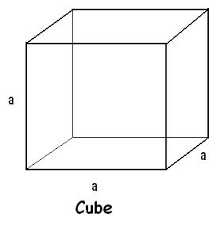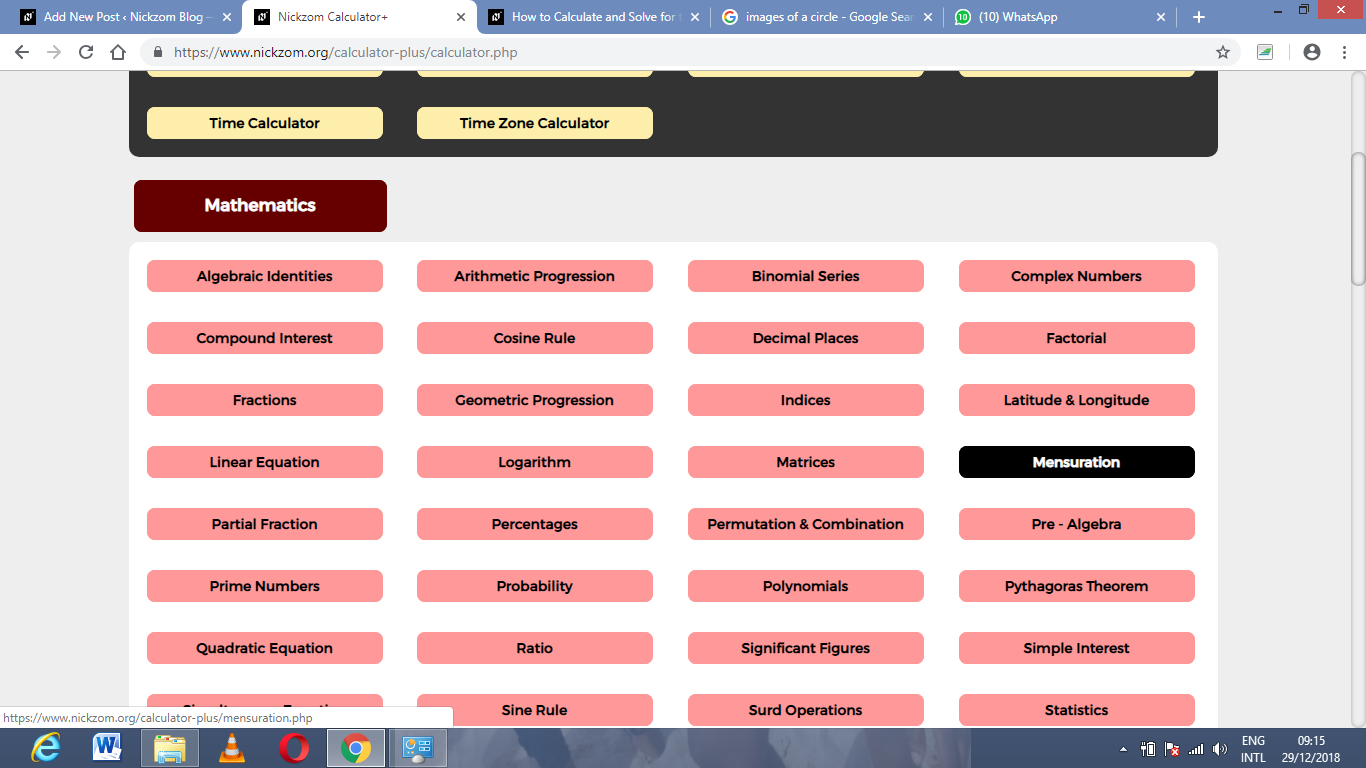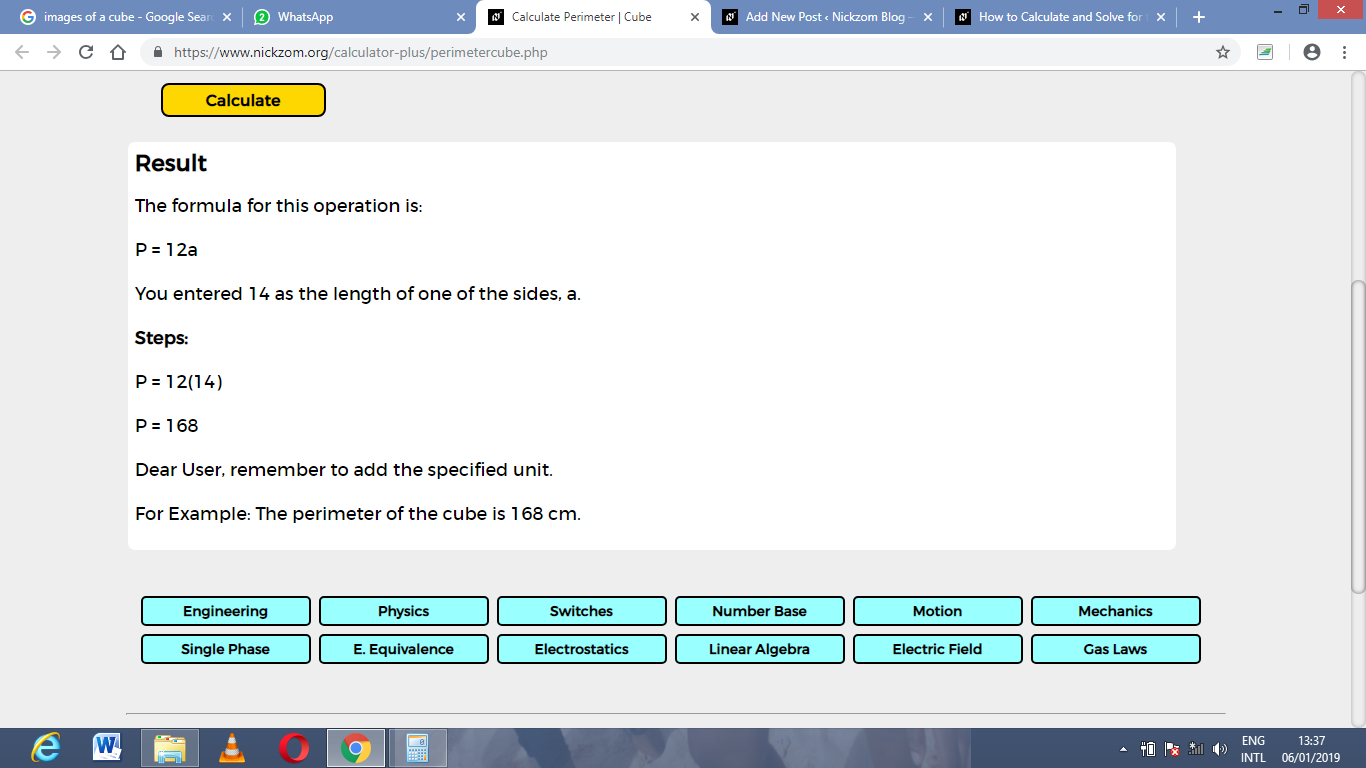# How to Calculate and Solve for the Length of a Side and Perimeter of a Cube | The Calculator EncyclopediaThe image above is a cube.

To compute the perimeter of a cube, one essential parameter is needed and this parameter is length of one of the sides (a).

The formula for calculating the perimeter of a cube:

P = 12a

Where;
P = Perimeter of the cube
a = Length of one of the sides of the cube

Let’s solve an example;
Find the perimeter of a cube when the length of one of the sides is 14 cm.

This implies that;
a = Length of one of the sides = 14 cm

P = 12a
P = 12 x 14
P = 168

Therefore, the perimeter of the cube is 168 cm.

Calculating the Length of one of the sides of a Cube using the Perimeter of the Cube.

a = P / 12

Where;
a = Length of one of the sides of the cube
P = Perimeter of the cube

Let’s solve an example;
Find the length of one of the sides of a cube when the perimeter of the cube is 200 cm.

This implies that;
P = Perimeter of the cube = 200 cm

a = P / 12
a = 200 / 12
a = 16.67

Therefore, the length of one of the sides is 16.67 cm.

Nickzom Calculator – The Calculator Encyclopedia is capable of calculating the perimeter of a cube.

To get the answer and workings of the perimeter of a cube using the Nickzom Calculator – The Calculator Encyclopedia. First, you need to obtain the app.

You can get this app via any of these means:

To get access to the professional version via web, you need to register and subscribe for NGN 1,500 per annum to have utter access to all functionalities.
You can also try the demo version via https://www.nickzom.org/calculator

Once, you have obtained the calculator encyclopedia app, proceed to the Calculator Map, then click on Mensuration under the Mathematics sectionNow, click on Perimeter of a cube under MensurationThe screenshot below displays the page or activity to enter your value, to get the answer for the perimeter of a cube according to the respective parameter which is the length of one of the sides (a).Now, enter the values appropriately and accordingly for the parameter as required by the example above where the length of one of the sides (a) is 14 cm.Finally, Click on CalculateAs you can see from the screenshot above, Nickzom Calculator – The Calculator Encyclopedia solves for the perimeter of a cube and presents the formula, workings and steps too.